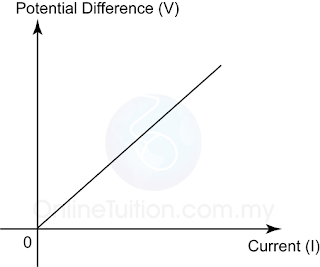# Ohm's Law - Relationship Between Current and Potential Difference

### Ohm’s Law1. The relationship between the current passing through 2 points in a conductor and the potential difference between the 2 points is given by Ohm's law.
2. Ohm’s Law states that the current flowing in the metallic conductor is directly proportional to the potential difference applied across it’s ends, provided that the physical conditions ( such as temperature ) are constant. $IαV$ or $V=kI$ where k is a constant

Example:
What is the current flow through an 800Ω toaster when it is operating on 240V?

$R= V I I= V R = 240 800 =0.3A$